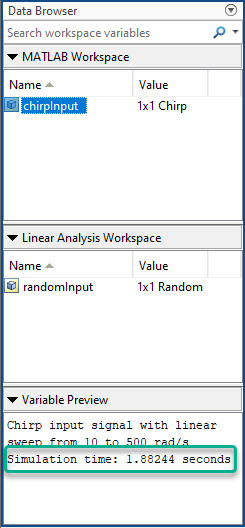# getSimulationTime

Final time of simulation for frequency response estimation

## Syntax

``tfinal = getSimulationTime(input)``

## Description

example

````tfinal = getSimulationTime(input)` returns the final time of the Simulink® simulation performed during frequency response estimation using the input signal `input`. Altering `input` to reduce the final simulation time can help reduce the time it takes to perform frequency response estimation.```

## Examples

collapse all

Create a sinestream input signal.

```input = frest.Sinestream('Amplitude',1e-3,... 'Frequency',logspace(1,3,50),... 'SamplesPerPeriod',40,'FreqUnits','Hz');```

The sinestream signal `input` includes 50 frequencies spaced logarithmically between 10 Hz and 1000 Hz. Each frequency is sampled 40 times per period.

Calculate the final simulation time of an estimation using that signal.

`tfinal = getSimulationTime(input)`
```tfinal = 4.4186 ```

`tfinal` indicates that frequency response estimation of any model with this input signal would simulate the model for 4.4186 s.

## Input Arguments

collapse all

Input signal for frequency response estimation with the `frestimate` function, specified as one of the following objects.

You can create input signals at the command line or export signals that you create using the Model Linearizer app.

## Output Arguments

collapse all

Final time for a simulation performed during frequency response estimation using the specified input signal, returned as a scalar value.

## Alternative Functionality

### App

You can view the simulation time for an input signal when estimating a frequency response using the Model Linearizer app. To do so, in the MATLAB Workspace or Linear Analysis Workspace section, select the input signal. The app shows the simulation time in the Variable Preview section.## Version History

Introduced in R2012a